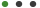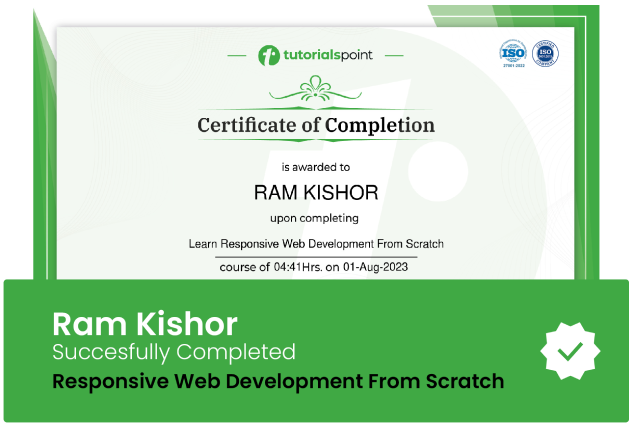### Easy Statistics: Linear and Non-Linear RegressionF Buscha

# Easy Statistics: Linear and Non-Linear Regression

An easy introduction to Ordinary Least Squares, Logit and Probit regression methodsUpdated on Sep, 2023Language - EnglishF BuschaStatistics,Business,Finance & Accounting,Regression Analysis30-days Money-Back Guarantee

Training 5 or more people ?

## Course Description

Learning and applying new statistical techniques can often be a daunting experience.

"Easy Statistics" is designed to provide you with a compact, and easy to understand, course that focuses on the basic principles of statistical methodology.

This course will focus on the concept of linear regression, non-linear regression and regression modelling. Specifically Ordinary Least Squares, Logit and Probit Regression.

The first two parts will explain what regression is and how linear and non-liner regression works. It will examine how Ordinary Least Squares (OLS) works and how Logit and Probit models work. It will do this without any complicated equations or mathematics. The focus of this course is on application and interpretation of regression. The learning on this course is underpinned by animated graphics that demonstrate particular statistical concepts.

No prior knowledge is necessary and this course is for anyone who needs to engage with quantitative analysis.

The main learning outcomes are:

1. To learn and understand the basic statistical intuition behind Ordinary Least Squares

2. To be at ease with general regression terminology and the assumptions behind Ordinary Least Squares

3. To be able to comfortably interpret and analyze complicated linear regression output from Ordinary Least Squares

4. To learn tips and tricks around linear regression analysis

5. To learn and understand the basic statistical intuition behind non-linear regression

6. To learn and understand how Logit and Probit models work

7. To be able to comfortably interpret and analyze complicated regression output from Logit and Probit regression

8. To learn tips and tricks around non-linear Regression analysis

Specific topics that will be covered are:

• What kinds of regression analysis exist

• Correlation versus causation

• Parametric and non-parametric lines of best fit

• The least squares method

• R-squared

• Beta's, standard errors

• T-statistics, p-values and confidence intervals

• Best Linear Unbiased Estimator

• The Gauss-Markov assumptions

• Bias versus efficiency

• Homoskedasticity

• Collinearity

• Functional form

• Zero conditional mean

• Regression in logs

• Practical model building

• Understanding regression output

• Presenting regression output

• What kinds of non-linear regression analysis exist

• How does non-linear regression work?

• Why is non-linear regression useful?

• What is Maximum Likelihood?

• The Linear Probability Model

• Logit and Probit regression

• Latent variables

• Marginal effects

• Dummy variables in Logit and Probit regression

• Goodness-of-fit statistics

• Odd-ratios for Logit models

• Practical Logit and Probit model building in Stata

The computer software Stata will be used to demonstrate practical examples.

### Goals

What will you learn in this course:

• To learn the theory behind linear and non-linear regression analysis.
• To be at ease with regression terminology.
• To learn the assumptions and requirements of Ordinary Least Squares (OLS) regression.
• To comfortably interpret and analyse regression output from Ordinary Least Squares.
• To learn and understand how Logit and Probit models work.
• To learn tips and tricks around Non-Linear Regression analysis.
• Practical examples

### Prerequisites

What are the prerequisites for this course?

• None other than an interest in Statistics and Regression.## Curriculum

Check out the detailed breakdown of what’s inside the course

Linear Regression
27 Lectures
•What is Easy Statistics: Linear Regression? 01:17 01:17
•What is Regression? 01:08 01:08
•Learning Outcomes 00:38 00:38
•Who is this Course for? 00:42 00:42
•Pre-requisites 00:48 00:48
•Using Stata 01:01 01:01
•What is Regression Analysis? 02:45 02:45
•What is Linear Regression? 01:47 01:47
•Why is Regression Analysis Useful? 01:36 01:36
•What Types of Regression Analysis Exist? 02:33 02:33
•Explaining Regression 03:40 03:40
•Lines of Best Fit 07:58 07:58
•Causality vs Correlation 01:55 01:55
•What is Ordinary Least Squares? 01:04 01:04
•Ordinary Least Squares Visual 1 04:15 04:15
•Ordinary Least Squares Visual 2 07:39 07:39
•Sum of Squares 03:13 03:13
•Best Linear Unbiased Estimator 04:44 04:44
•The Gauss-Markov Assumptions 00:41 00:41
•Homoskedasticity 02:13 02:13
•No Perfect Collinearity 02:35 02:35
•Linear in Parameters 02:43 02:43
•Zero Conditional Mean 02:15 02:15
•How to Test and Correct for Endogeneity 00:52 00:52
•The Gauss-Markov Assumptions Recap 01:56 01:56
•Stata - Applied Examples 21:32 21:32
•Final Thoughts and Tips 03:55 03:55
Non-Linear Regression
22 Lectures## Instructor DetailsF Buscha

Franz is a Professor of Economics at the University of Westminster. Franz joined the University of Westminster in 2006 after completing his PhD in Economics at Lancaster University.

Franz's personal research interests are in education economics, labor economics, and applied econometrics. Franz has made scientific contributions to issues such as social mobility, measuring the returns to education, the effect of weather of happiness and identity formation. He has been involved in numerous funded research projects from research councils and government departments.

Franz has contributed to wide range of projects including policy evaluation and bespoke econometric advice to UK government departments. These include the Ministry of Defence, HM Revenue and Customs, the Department for Education and the Department for Business, Innovation and Skills.

He has published in leading journals such as Economics of Education Review, the Oxford Bulletin of Economics and Statistics, the British Journal of Political Science and the British Journal of Sociology. Franz has also contributed to numerous policy reports and his research has been covered by media outlets such as BBC news, BBC Radio 4, The Economist, The Guardian, The Times, and Huffington Post. Franz also has a monthly radio program called Policy Matters on Share Radio.

## Course Certificate

User your certification to make a career change or to advance in your current career. Salaries are among the highest in the world.